Скачать презентацию Chapter 22 Questions and Problems

5d9cf7c7024a23ba17315c4004648ba3.ppt

• Количество слайдов: 31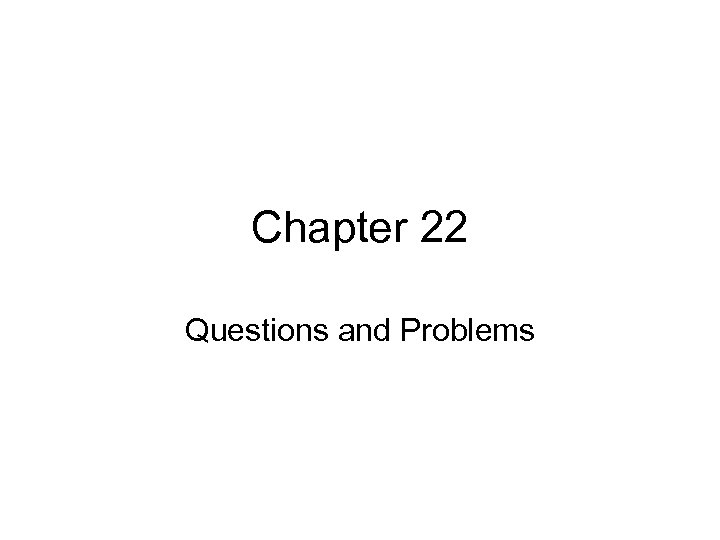Chapter 22 Questions and Problems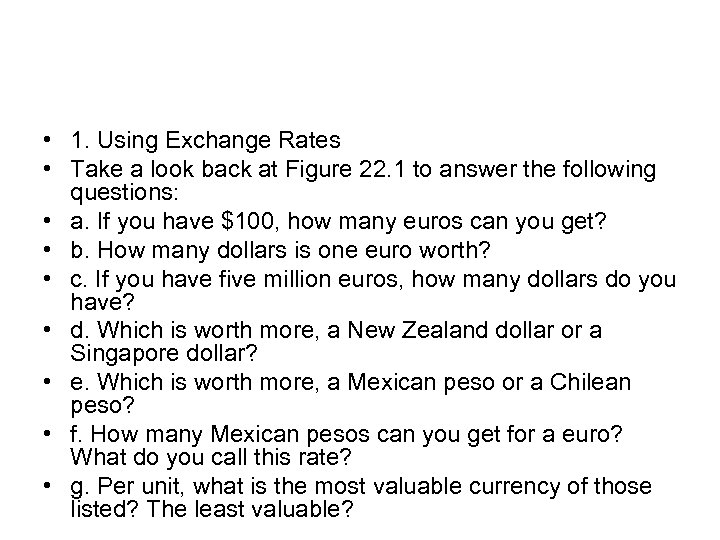• 1. Using Exchange Rates • Take a look back at Figure 22. 1 to answer the following questions: • a. If you have \$100, how many euros can you get? • b. How many dollars is one euro worth? • c. If you have five million euros, how many dollars do you have? • d. Which is worth more, a New Zealand dollar or a Singapore dollar? • e. Which is worth more, a Mexican peso or a Chilean peso? • f. How many Mexican pesos can you get for a euro? What do you call this rate? • g. Per unit, what is the most valuable currency of those listed? The least valuable?• 1. • • • Using the quotes from the table, we get: a. b. c. d. e. f. g. \$100(€ 0. 8206/\$1) = € 82. 06 \$1. 2186 € 5 M(\$1. 2186/€) = \$6, 093, 103 Singapore dollar Mexican peso (P 11. 4850/\$1)(\$1. 2186/€ 1) = P 13. 9959/€ This is a cross rate. Most valuable: Bahrain dinar = \$3. 3920 Least valuable: Turkish lira = \$0. 00000067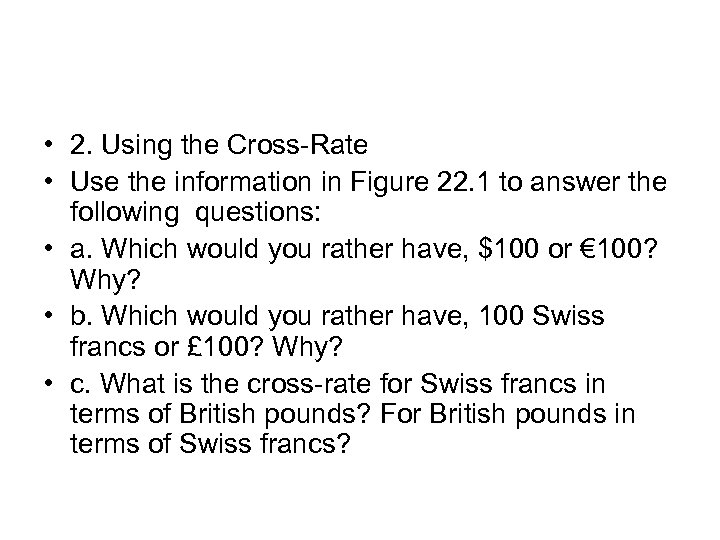• 2. Using the Cross-Rate • Use the information in Figure 22. 1 to answer the following questions: • a. Which would you rather have, \$100 or € 100? Why? • b. Which would you rather have, 100 Swiss francs or £ 100? Why? • c. What is the cross-rate for Swiss francs in terms of British pounds? For British pounds in terms of Swiss francs?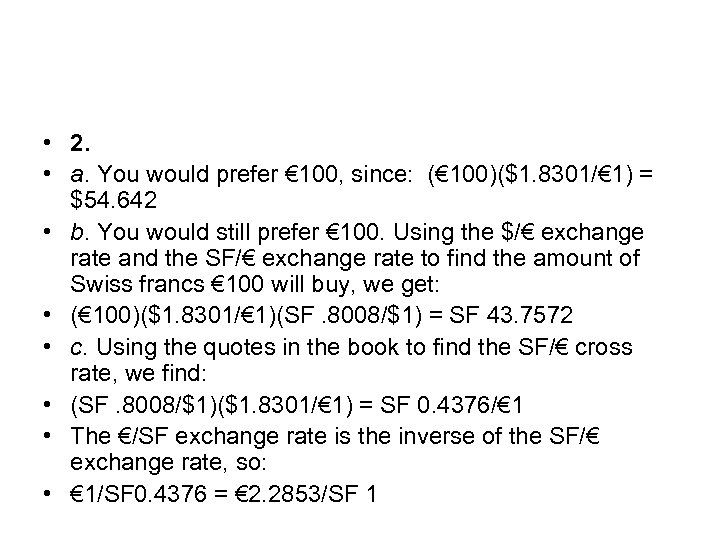• 2. • a. You would prefer € 100, since: (€ 100)(\$1. 8301/€ 1) = \$54. 642 • b. You would still prefer € 100. Using the \$/€ exchange rate and the SF/€ exchange rate to find the amount of Swiss francs € 100 will buy, we get: • (€ 100)(\$1. 8301/€ 1)(SF. 8008/\$1) = SF 43. 7572 • c. Using the quotes in the book to find the SF/€ cross rate, we find: • (SF. 8008/\$1)(\$1. 8301/€ 1) = SF 0. 4376/€ 1 • The €/SF exchange rate is the inverse of the SF/€ exchange rate, so: • € 1/SF 0. 4376 = € 2. 2853/SF 1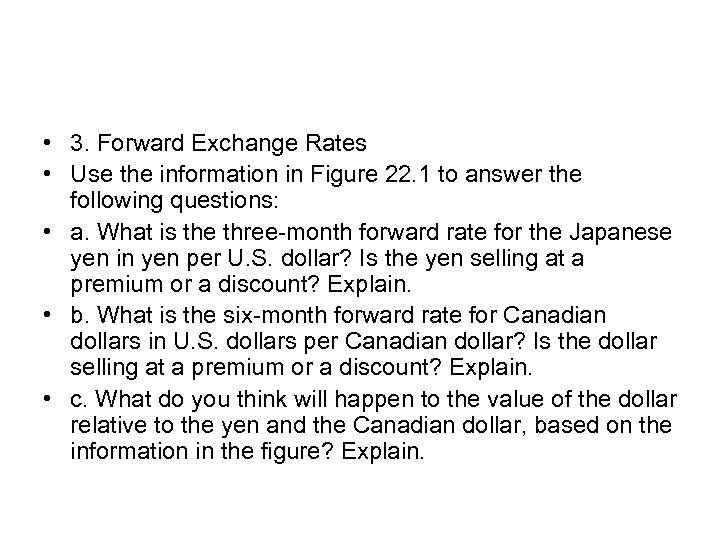• 3. Forward Exchange Rates • Use the information in Figure 22. 1 to answer the following questions: • a. What is the three-month forward rate for the Japanese yen in yen per U. S. dollar? Is the yen selling at a premium or a discount? Explain. • b. What is the six-month forward rate for Canadian dollars in U. S. dollars per Canadian dollar? Is the dollar selling at a premium or a discount? Explain. • c. What do you think will happen to the value of the dollar relative to the yen and the Canadian dollar, based on the information in the figure? Explain.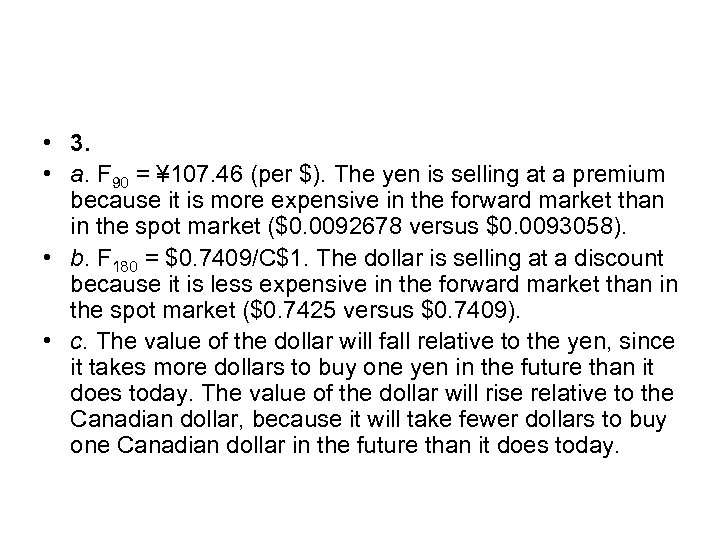• 3. • a. F 90 = ¥ 107. 46 (per \$). The yen is selling at a premium because it is more expensive in the forward market than in the spot market (\$0. 0092678 versus \$0. 0093058). • b. F 180 = \$0. 7409/C\$1. The dollar is selling at a discount because it is less expensive in the forward market than in the spot market (\$0. 7425 versus \$0. 7409). • c. The value of the dollar will fall relative to the yen, since it takes more dollars to buy one yen in the future than it does today. The value of the dollar will rise relative to the Canadian dollar, because it will take fewer dollars to buy one Canadian dollar in the future than it does today.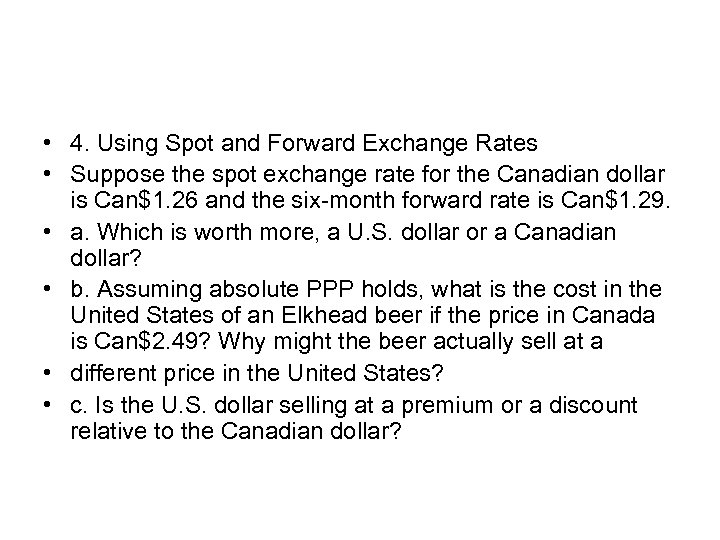• 4. Using Spot and Forward Exchange Rates • Suppose the spot exchange rate for the Canadian dollar is Can\$1. 26 and the six-month forward rate is Can\$1. 29. • a. Which is worth more, a U. S. dollar or a Canadian dollar? • b. Assuming absolute PPP holds, what is the cost in the United States of an Elkhead beer if the price in Canada is Can\$2. 49? Why might the beer actually sell at a • different price in the United States? • c. Is the U. S. dollar selling at a premium or a discount relative to the Canadian dollar?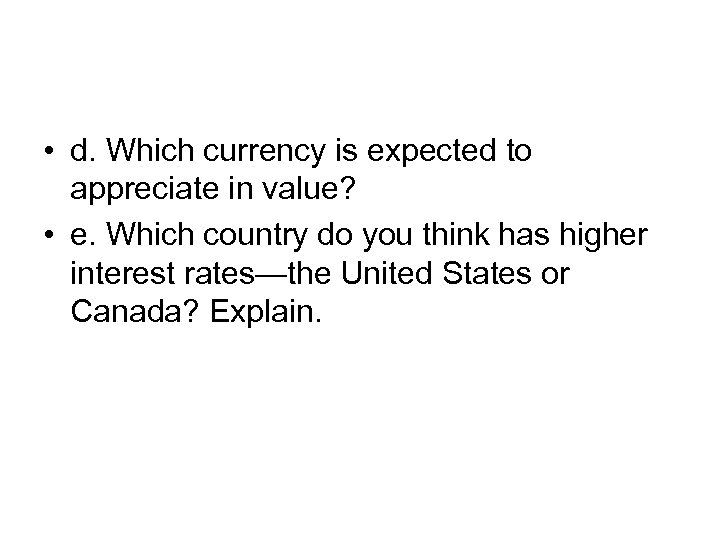• d. Which currency is expected to appreciate in value? • e. Which country do you think has higher interest rates—the United States or Canada? Explain.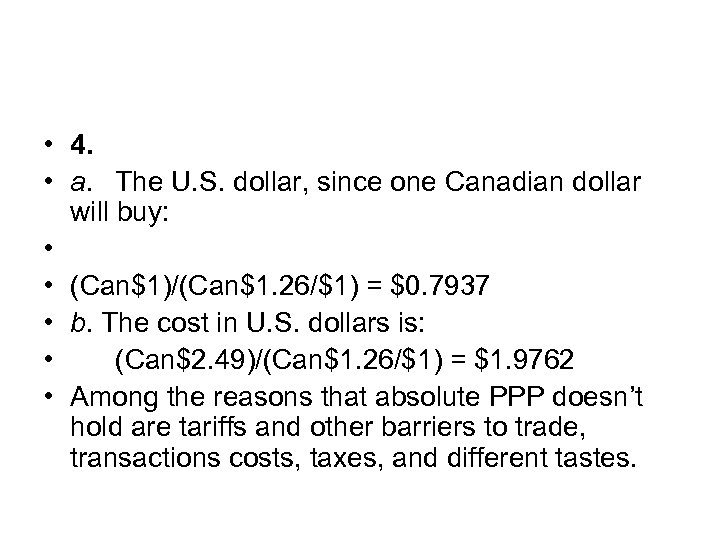• 4. • a. The U. S. dollar, since one Canadian dollar will buy: • • (Can\$1)/(Can\$1. 26/\$1) = \$0. 7937 • b. The cost in U. S. dollars is: • (Can\$2. 49)/(Can\$1. 26/\$1) = \$1. 9762 • Among the reasons that absolute PPP doesn’t hold are tariffs and other barriers to trade, transactions costs, taxes, and different tastes.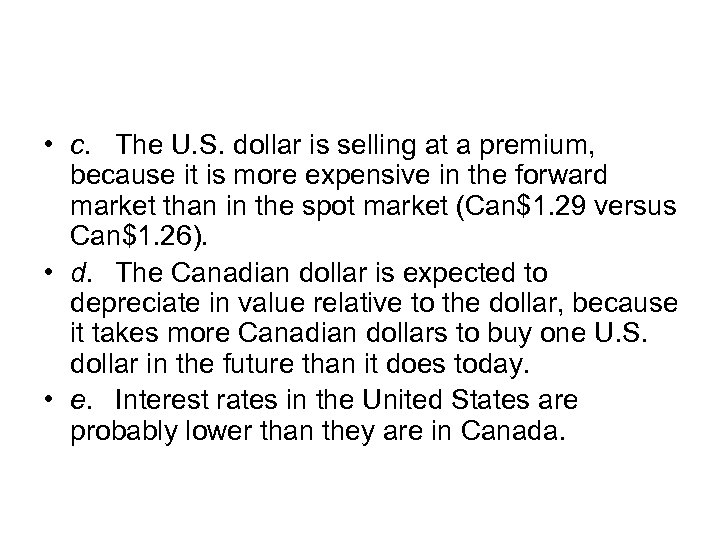• c. The U. S. dollar is selling at a premium, because it is more expensive in the forward market than in the spot market (Can\$1. 29 versus Can\$1. 26). • d. The Canadian dollar is expected to depreciate in value relative to the dollar, because it takes more Canadian dollars to buy one U. S. dollar in the future than it does today. • e. Interest rates in the United States are probably lower than they are in Canada.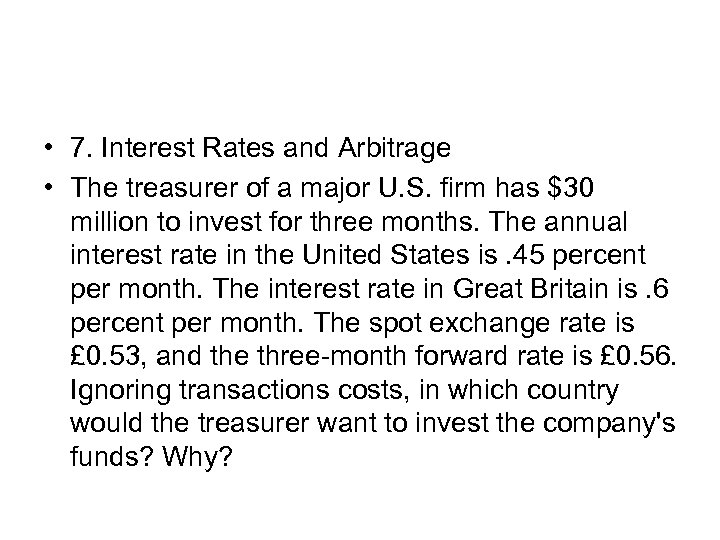• 7. Interest Rates and Arbitrage • The treasurer of a major U. S. firm has \$30 million to invest for three months. The annual interest rate in the United States is. 45 percent per month. The interest rate in Great Britain is. 6 percent per month. The spot exchange rate is £ 0. 53, and the three-month forward rate is £ 0. 56. Ignoring transactions costs, in which country would the treasurer want to invest the company's funds? Why?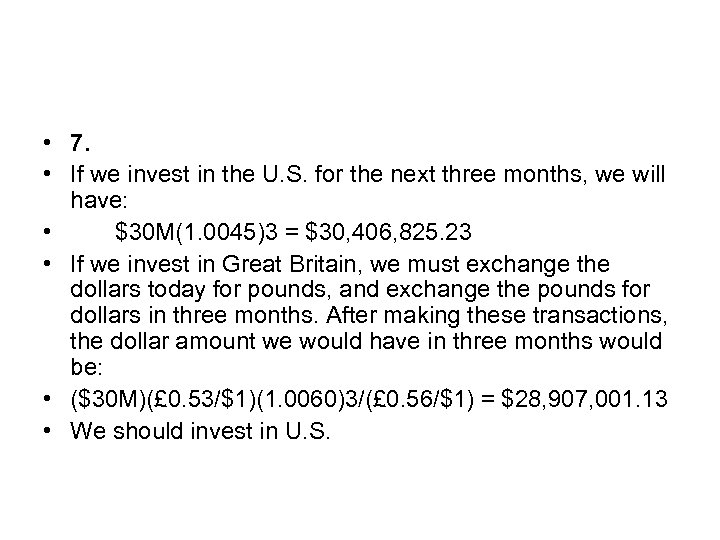• 7. • If we invest in the U. S. for the next three months, we will have: • \$30 M(1. 0045)3 = \$30, 406, 825. 23 • If we invest in Great Britain, we must exchange the dollars today for pounds, and exchange the pounds for dollars in three months. After making these transactions, the dollar amount we would have in three months would be: • (\$30 M)(£ 0. 53/\$1)(1. 0060)3/(£ 0. 56/\$1) = \$28, 907, 001. 13 • We should invest in U. S.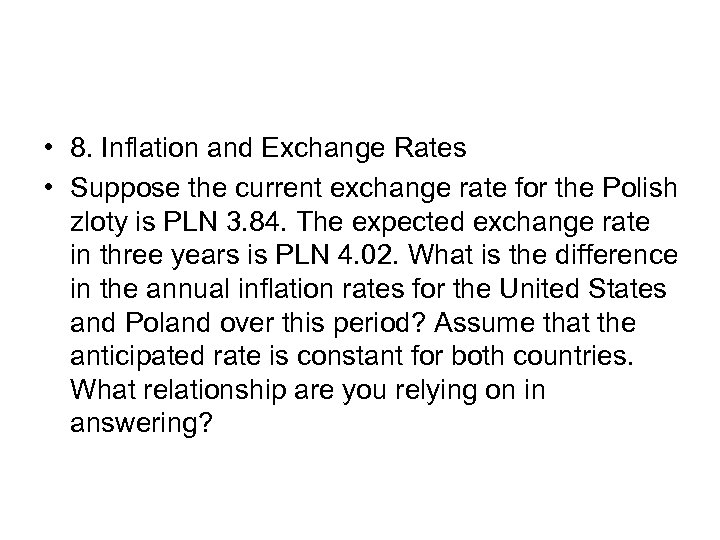• 8. Inflation and Exchange Rates • Suppose the current exchange rate for the Polish zloty is PLN 3. 84. The expected exchange rate in three years is PLN 4. 02. What is the difference in the annual inflation rates for the United States and Poland over this period? Assume that the anticipated rate is constant for both countries. What relationship are you relying on in answering?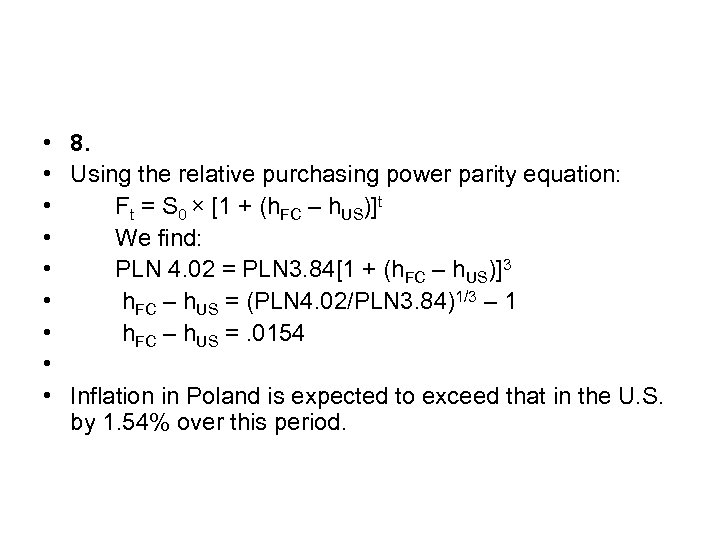• 8. • Using the relative purchasing power parity equation: • Ft = S 0 × [1 + (h. FC – h. US)]t • We find: • PLN 4. 02 = PLN 3. 84[1 + (h. FC – h. US)]3 • h. FC – h. US = (PLN 4. 02/PLN 3. 84)1/3 – 1 • h. FC – h. US =. 0154 • • Inflation in Poland is expected to exceed that in the U. S. by 1. 54% over this period.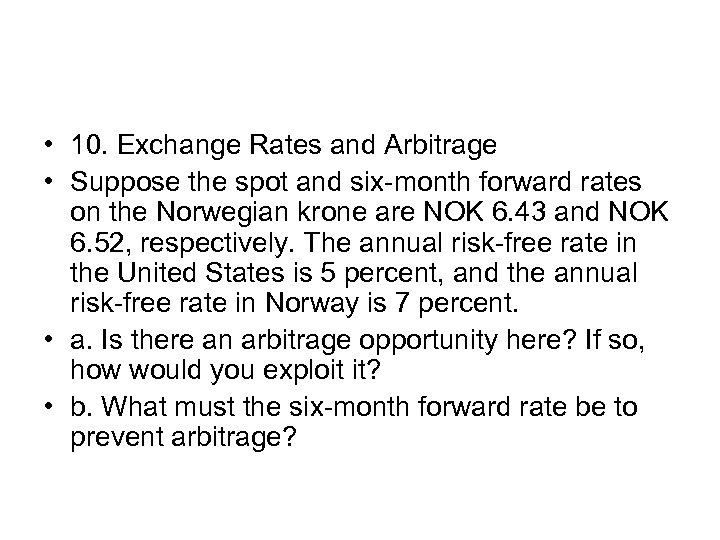• 10. Exchange Rates and Arbitrage • Suppose the spot and six-month forward rates on the Norwegian krone are NOK 6. 43 and NOK 6. 52, respectively. The annual risk-free rate in the United States is 5 percent, and the annual risk-free rate in Norway is 7 percent. • a. Is there an arbitrage opportunity here? If so, how would you exploit it? • b. What must the six-month forward rate be to prevent arbitrage?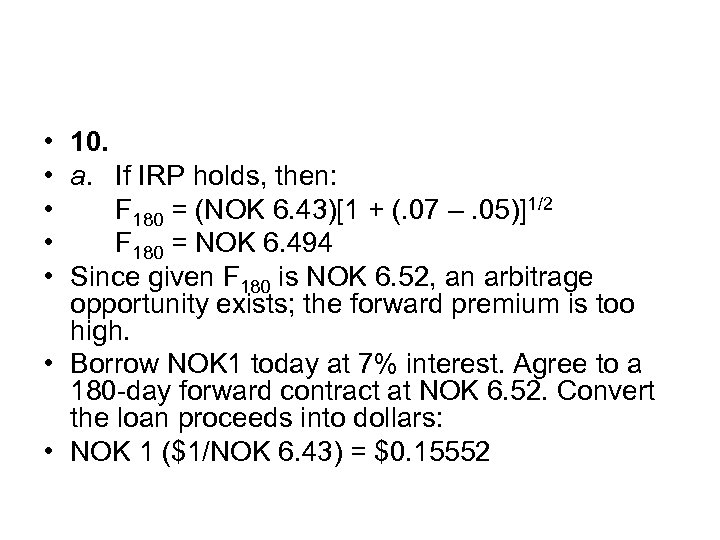• 10. • a. If IRP holds, then: • F 180 = (NOK 6. 43)[1 + (. 07 –. 05)]1/2 • F 180 = NOK 6. 494 • Since given F 180 is NOK 6. 52, an arbitrage opportunity exists; the forward premium is too high. • Borrow NOK 1 today at 7% interest. Agree to a 180 -day forward contract at NOK 6. 52. Convert the loan proceeds into dollars: • NOK 1 (\$1/NOK 6. 43) = \$0. 15552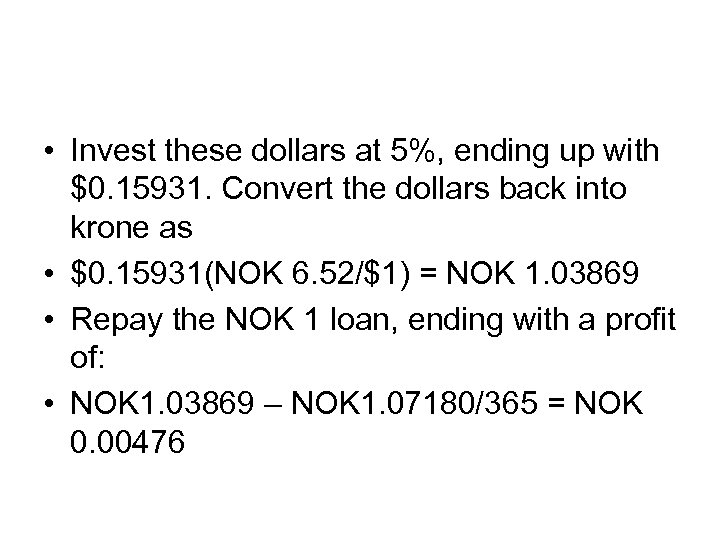• Invest these dollars at 5%, ending up with \$0. 15931. Convert the dollars back into krone as • \$0. 15931(NOK 6. 52/\$1) = NOK 1. 03869 • Repay the NOK 1 loan, ending with a profit of: • NOK 1. 03869 – NOK 1. 07180/365 = NOK 0. 00476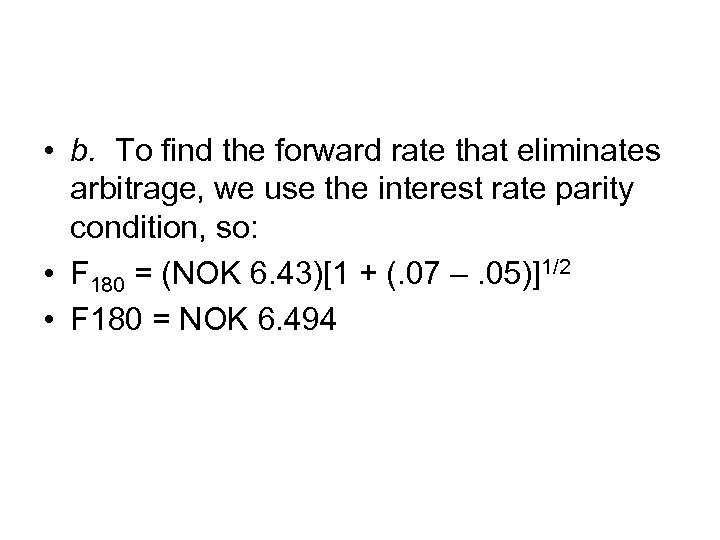• b. To find the forward rate that eliminates arbitrage, we use the interest rate parity condition, so: • F 180 = (NOK 6. 43)[1 + (. 07 –. 05)]1/2 • F 180 = NOK 6. 494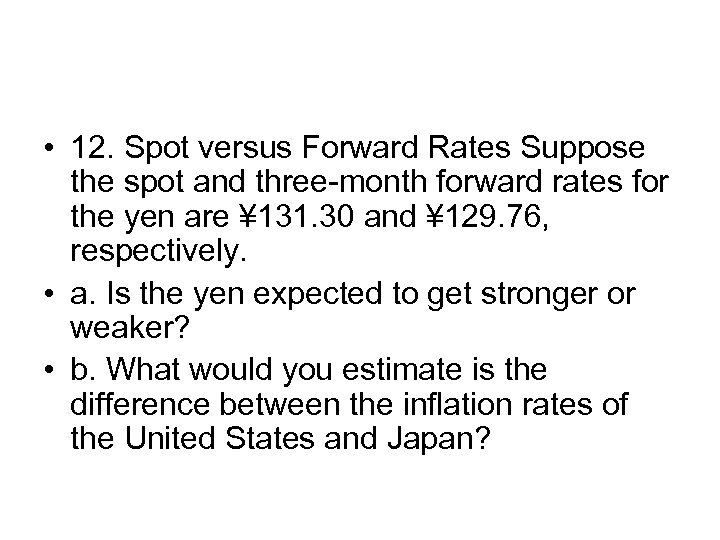• 12. Spot versus Forward Rates Suppose the spot and three-month forward rates for the yen are ¥ 131. 30 and ¥ 129. 76, respectively. • a. Is the yen expected to get stronger or weaker? • b. What would you estimate is the difference between the inflation rates of the United States and Japan?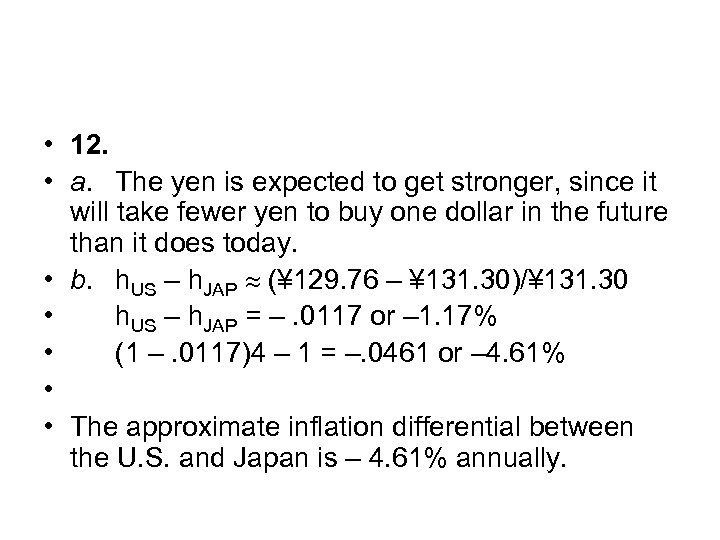• 12. • a. The yen is expected to get stronger, since it will take fewer yen to buy one dollar in the future than it does today. • b. h. US – h. JAP (¥ 129. 76 – ¥ 131. 30)/¥ 131. 30 • h. US – h. JAP = –. 0117 or – 1. 17% • (1 –. 0117)4 – 1 = –. 0461 or – 4. 61% • • The approximate inflation differential between the U. S. and Japan is – 4. 61% annually.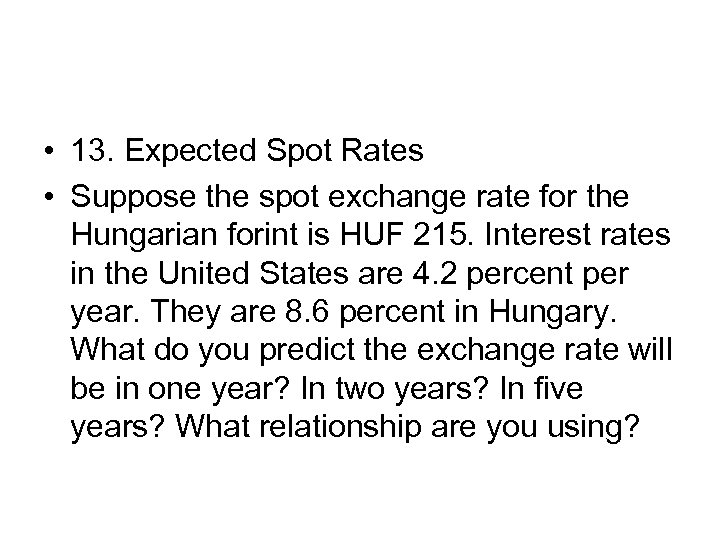• 13. Expected Spot Rates • Suppose the spot exchange rate for the Hungarian forint is HUF 215. Interest rates in the United States are 4. 2 percent per year. They are 8. 6 percent in Hungary. What do you predict the exchange rate will be in one year? In two years? In five years? What relationship are you using?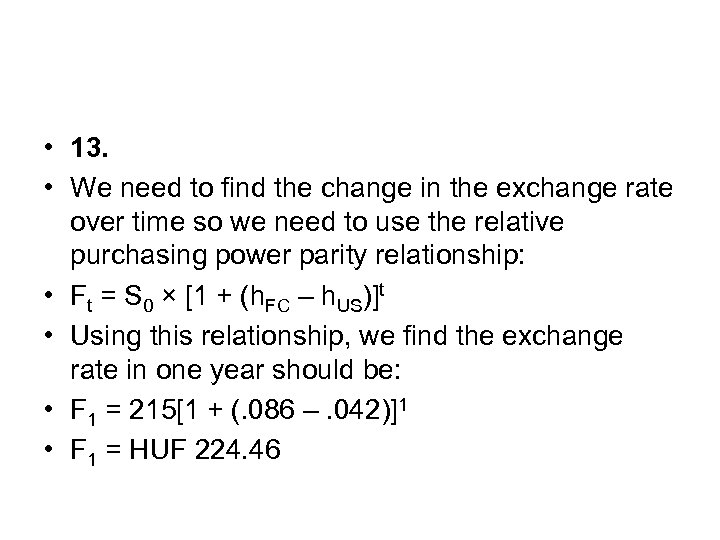• 13. • We need to find the change in the exchange rate over time so we need to use the relative purchasing power parity relationship: • Ft = S 0 × [1 + (h. FC – h. US)]t • Using this relationship, we find the exchange rate in one year should be: • F 1 = 215[1 + (. 086 –. 042)]1 • F 1 = HUF 224. 46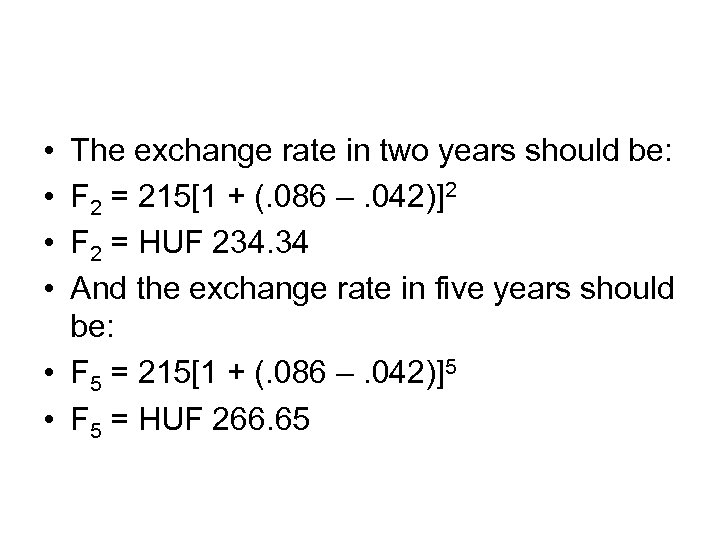• • The exchange rate in two years should be: F 2 = 215[1 + (. 086 –. 042)]2 F 2 = HUF 234. 34 And the exchange rate in five years should be: • F 5 = 215[1 + (. 086 –. 042)]5 • F 5 = HUF 266. 65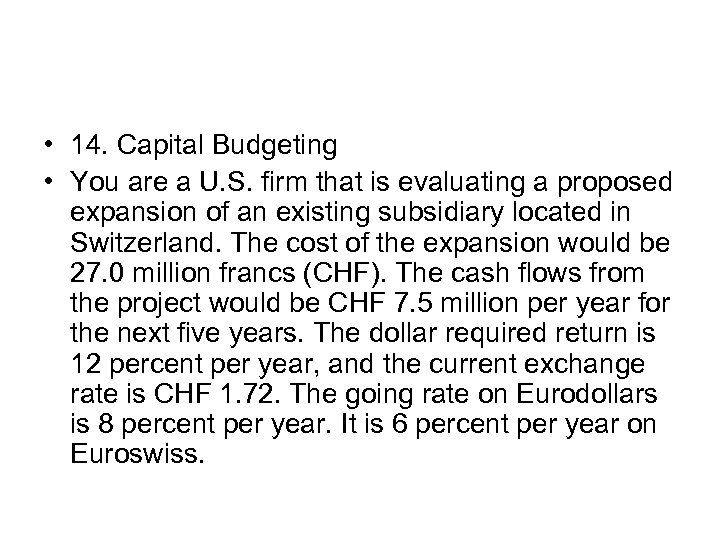• 14. Capital Budgeting • You are a U. S. firm that is evaluating a proposed expansion of an existing subsidiary located in Switzerland. The cost of the expansion would be 27. 0 million francs (CHF). The cash flows from the project would be CHF 7. 5 million per year for the next five years. The dollar required return is 12 percent per year, and the current exchange rate is CHF 1. 72. The going rate on Eurodollars is 8 percent per year. It is 6 percent per year on Euroswiss.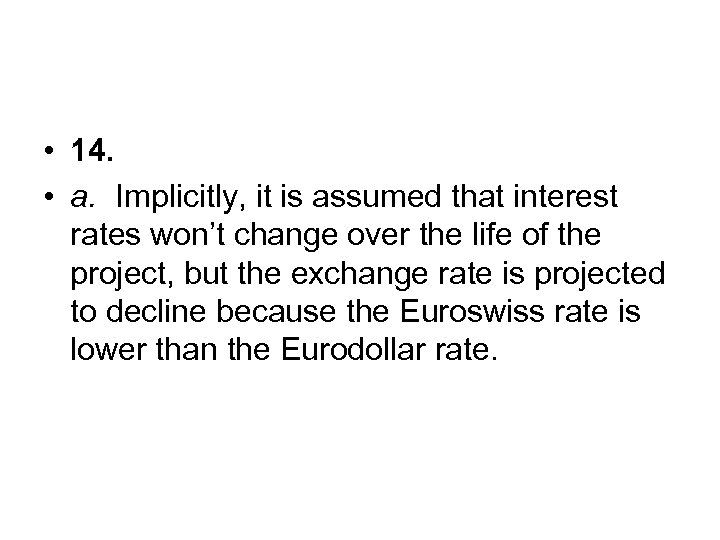• 14. • a. Implicitly, it is assumed that interest rates won’t change over the life of the project, but the exchange rate is projected to decline because the Euroswiss rate is lower than the Eurodollar rate.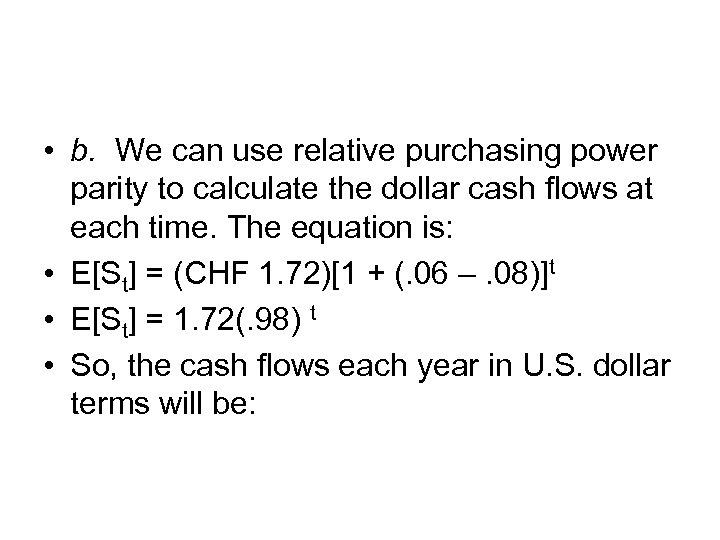• b. We can use relative purchasing power parity to calculate the dollar cash flows at each time. The equation is: • E[St] = (CHF 1. 72)[1 + (. 06 –. 08)]t • E[St] = 1. 72(. 98) t • So, the cash flows each year in U. S. dollar terms will be: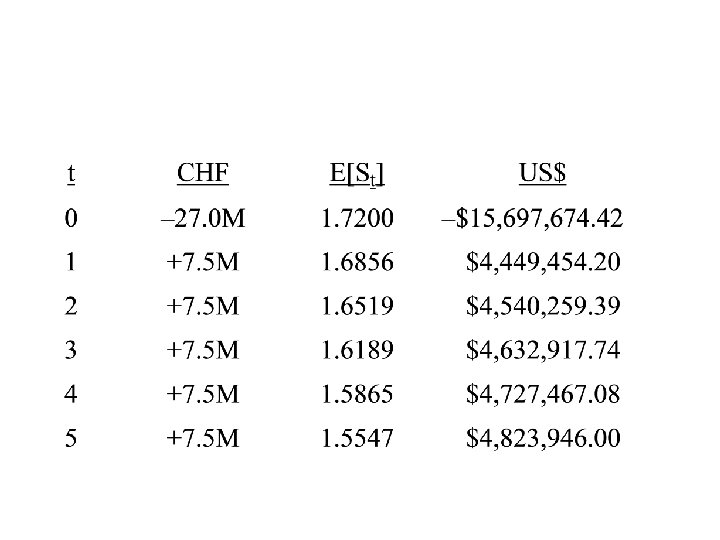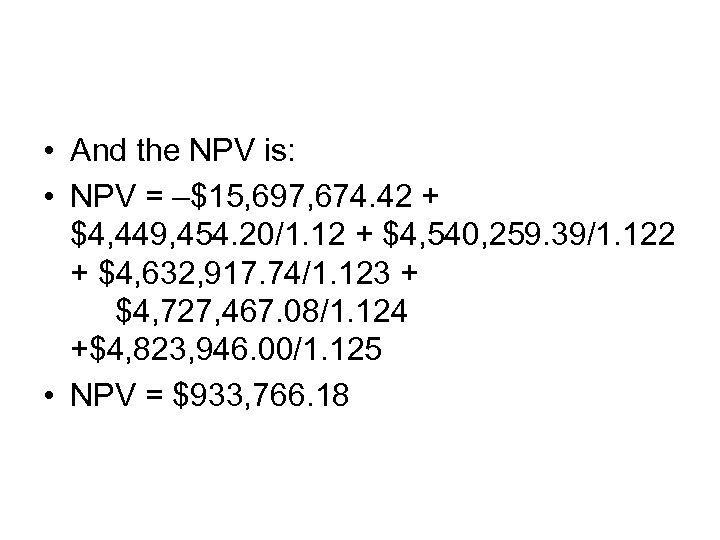• And the NPV is: • NPV = –\$15, 697, 674. 42 + \$4, 449, 454. 20/1. 12 + \$4, 540, 259. 39/1. 122 + \$4, 632, 917. 74/1. 123 + \$4, 727, 467. 08/1. 124 +\$4, 823, 946. 00/1. 125 • NPV = \$933, 766. 18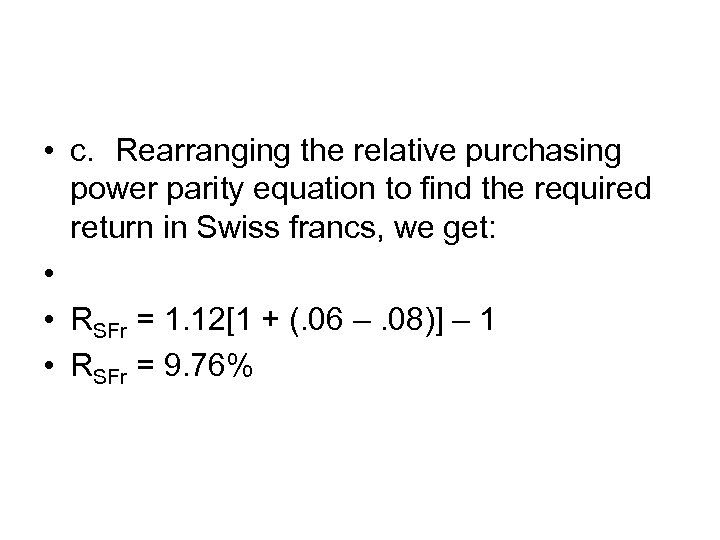• c. Rearranging the relative purchasing power parity equation to find the required return in Swiss francs, we get: • • RSFr = 1. 12[1 + (. 06 –. 08)] – 1 • RSFr = 9. 76%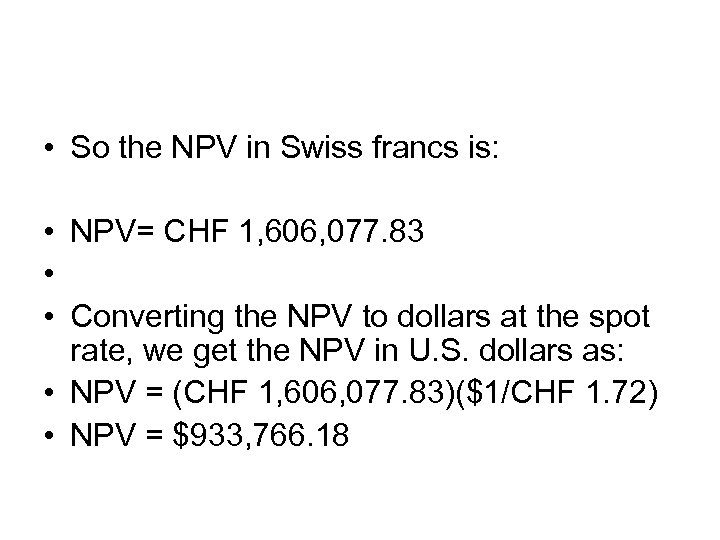• So the NPV in Swiss francs is: • NPV= CHF 1, 606, 077. 83 • • Converting the NPV to dollars at the spot rate, we get the NPV in U. S. dollars as: • NPV = (CHF 1, 606, 077. 83)(\$1/CHF 1. 72) • NPV = \$933, 766. 18